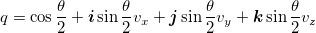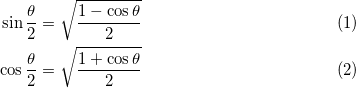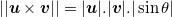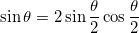lolengine.net Go back Open original

# Beautiful maths simplification: quaternion from two vectors

In this article I would like to explain the thought process behind the derivation of a widely used formula: finding a quaternion representing the rotation between two 3D vectors. Nothing really new, but hopefully a few ideas could be reused at other times.

Note: the routine presented here is incomplete on purpose. For a version that can be used in production code, see the next article instead, Quaternion from two vectors: the final version.

## Naive method

A rotation is best visualised using a rotation axis and an angle. Except in degenerate cases, the rotation axis can be obtained by computing the cross product of the two original vectors:Then the angle can be obtained using the properties of the cross product and/or the dot product:Since θ is always between 0 and π, we only care about the dot product. This gives us some obvious code to create the quaternion (omitting corner cases such as θ = 0 for clarity):

quat quat::fromtwovectors(vec3 u, vec3 v)
{ float cos_theta = dot(normalize(u), normalize(v)); float angle = acos(cos_theta); vec3 w = normalize(cross(u, v)); return quat::fromaxisangle(angle, w);
}


This is naive but it works. Googling for “quaternion from two vectors” shows this forum post and this SO question where this method or a variation thereof is suggested.

## Looking under the hood

Let’s have a look at what happens when building the quaternion from an axis and an angle:This means the code for quat::fromaxisangle would look somewhat like this:

quat quat::fromaxisangle(float angle, vec3 axis)
{ float half_sin = sin(0.5f * angle); float half_cos = cos(0.5f * angle); return quat(half_cos, half_sin * axis.x, half_sin * axis.y, half_sin * axis.z);
}


## Avoiding trigonometry

If you read Iñigo Quilez’s recent article about avoiding trigonometry you’ll have probably frowned at the fact that we computed θ from cos(θ), then computed sin(θ/2) and cos(θ/2).

Indeed, it happens that there is a much simpler way to do it; the half-angle formulas from precalculus tell us the following:This allows us to simplify our quaternion creation code:

quat quat::fromtwovectors(vec3 u, vec3 v)
{ float cos_theta = dot(normalize(u), normalize(v)); float half_cos = sqrt(0.5f * (1.f + cos_theta)); float half_sin = sqrt(0.5f * (1.f - cos_theta)); vec3 w = normalize(cross(u, v)); return quat(half_cos, half_sin * w.x, half_sin * w.y, half_sin * w.z);
}


This is pretty nice. By using well known trigonometry formulas, we got rid of all trigonometry function calls!

## Avoiding square roots

It happens that we can do slightly better. Note that we normalize three vectors: u, v and cross(u, v). That’s three square roots. The thing is, we already know the norm of w through this formula:And we know sin(θ) from precalculus again:Also, using the fact that sqrt(a)sqrt(b) = sqrt(ab) lets us perform one less square root.

We can therefore come up with the following performance improvement:

quat quat::fromtwovectors(vec3 u, vec3 v)
{ float norm_u_norm_v = sqrt(sqlength(u) * sqlength(v)); float cos_theta = dot(u, v) / norm_u_norm_v; float half_cos = sqrt(0.5f * (1.f + cos_theta)); float half_sin = sqrt(0.5f * (1.f - cos_theta)); vec3 w = cross(u, v) / (norm_u_norm_v * 2.f * half_sin * half_cos); return quat(half_cos, half_sin * w.x, half_sin * w.y, half_sin * w.z);
}


Oh wait! We divide by sin(θ/2) to compute w, then we multiply by sin(θ/2) again. This means we don’t even need that variable, and we can simplify even further:

quat quat::fromtwovectors(vec3 u, vec3 v)
{ float norm_u_norm_v = sqrt(sqlength(u) * sqlength(v)); float cos_theta = dot(u, v) / norm_u_norm_v; float half_cos = sqrt(0.5f * (1.f + cos_theta)); vec3 w = cross(u, v) / (norm_u_norm_v * 2.f * half_cos); return quat(half_cos, w.x, w.y, w.z);
}


This is more or less the code used by the Ogre3D engine in OgreVector3.h, except they perform an additional normalisation step on the final result. This is mathematically useless, but due to numerical stability issues, it is probably safe to do so nonetheless.

This final normalisation step is actually an opportunity to simplify the code even further.

## Improving on Ogre3D

We are down to two square roots and four divisions, plus quite a few mul/adds. Depending on the platform that we are running on, it is possible to simplify even further and improve performance. For instance, on many SIMD architectures, normalising a quaternion can be very fast.

This is the code we get if we multiply every component of the quaternion by 2.f * half_cos and let normalize() do the rest of the job:

quat quat::fromtwovectors(vec3 u, vec3 v)
{ float norm_u_norm_v = sqrt(sqlength(u) * sqlength(v)); float cos_theta = dot(u, v) / norm_u_norm_v; float half_cos = sqrt(0.5f * (1.f + cos_theta)); vec3 w = cross(u, v) / norm_u_norm_v; return normalize(quat(2.f * half_cos * half_cos, w.x, w.y, w.z));
}


Now half_cos only appears in its squared form, and since it comes from a square root, we can simply omit that square root:

quat quat::fromtwovectors(vec3 u, vec3 v)
{ float norm_u_norm_v = sqrt(sqlength(u) * sqlength(v)); float cos_theta = dot(u, v) / norm_u_norm_v; vec3 w = cross(u, v) / norm_u_norm_v; return normalize(quat(1.f + cos_theta, w.x, w.y, w.z));
}


And using the same reasoning we can multiply every quaternion component by norm_u_norm_v:

quat quat::fromtwovectors(vec3 u, vec3 v)
{ float norm_u_norm_v = sqrt(sqlength(u) * sqlength(v)); vec3 w = cross(u, v); quat q = quat(norm_u_norm_v + dot(u, v), w.x, w.y, w.z); return normalize(q);
}


We are still doing two square roots and four divisions, some of which are hidden in normalize(), but the code is considerably shorter now.

## Final formIf u and v can be enforced to be unit vectors, norm_u_norm_v can be omitted and simply replaced with 1.0f:

quat quat::fromtwovectors(vec3 u, vec3 v)
{ vec3 w = cross(u, v); quat q = quat(1.f + dot(u, v), w.x, w.y, w.z); return normalize(q);
}


Isn’t it beautiful, considering the sin(), cos() and acos() ridden mess we started with?

This algorithm can be found all over the Internet, but I do not know who first came up with it. Also, a lot of 3D engines (both publicly available and slightly more private) could benefit from it.

In the comments below, Michael Norel provides the following improvement to the non-unit version. Since the values d = dot(u, v) and w = cross(u, v) are computed no matter what, the value sqlength(u) * sqlength(v) could be computed in a different way, *i.e.* d * d + sqlength(w). The following code does at least three multiplications less:

quat quat::fromtwovectors(vec3 u, vec3 v)
{ vec3 w = cross(u, v); quat q = quat(dot(u, v), w.x, w.y, w.z); q.w += length(q); return normalize(q);
}


Also, Marc B. Reynolds notes that, in the unit version, the final normalisation factor is sqrt((1 + dot(u, v))² + sqlength(cross(u, v))) which reduces to sqrt(2 + 2 dot(u, v)) thanks to the sin² + cos² identity. It leads to the following possibly improved version:

quat quat::fromtwovectors(vec3 u, vec3 v)
{ float m = sqrt(2.f + 2.f * dot(u, v)); vec3 w = (1.f / m) * cross(u, v); return quat(0.5f * m, w.x, w.y, w.z);
}